# Multiplying and Dividing Whole Numbers by 10, 100, and 1,000

Times up!
Times up! You may finish answering the current question and then proceed to your score!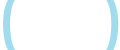Sorry, you have used all of your available hints for this lesson.
Practice Limit Reached

You've reached your daily practice limit of 25 questions.

Are you over 18 years old?
Award InformationAwarded toQuestion HintChoose Family Member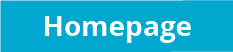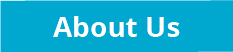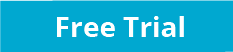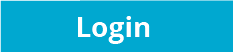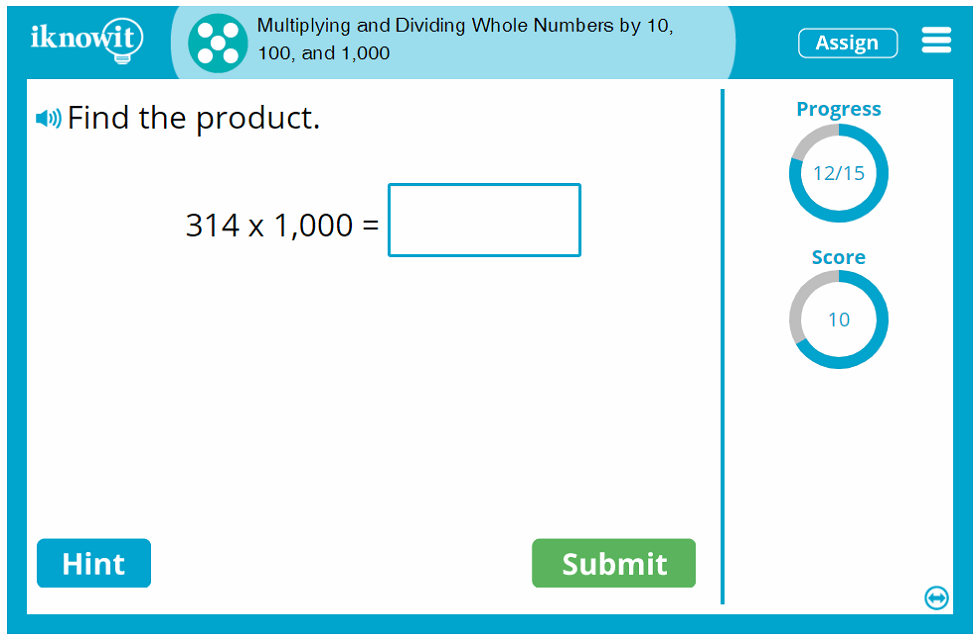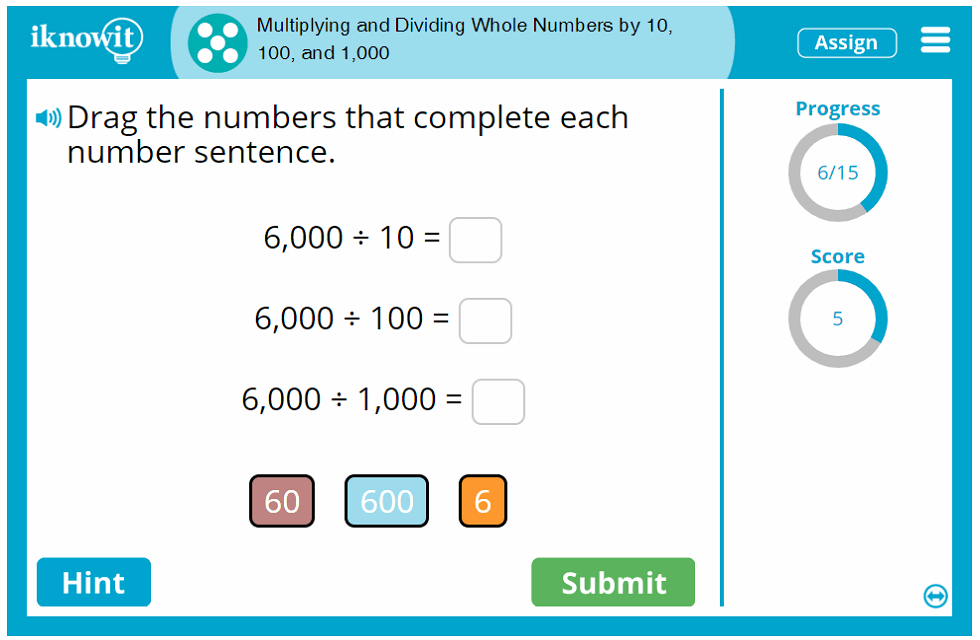## Interactive Math Game – Multiplying and Dividing Whole Numbers by 10, 100, and 1,000

Students will strengthen their basic multiplication and division skills in this fifth grade-level interactive math practice activity. Your class will practice multiplying and dividing whole numbers by 10, 100, and 1,000 in this online math game. Math questions are presented in a variety of formats, including drag-and-drop, fill-in-the-blank, and multiple choice.

If students need a little extra help solving one of the math problems in this interactive multiplication and division activity, they can click on the hint button. They will be shown a helpful clue: "To multiply a whole number by 10 – add one zero to the end of the number. To multiply a whole number by 100 – add two zeroes to the end of the number. To multiply a whole number by 1,000 – add three zeroes to the end of the number." Or alternately: "When dividing a whole number that ends in zero by: 10 – the quotient will have one less zero than the dividend; 100 – the quotient will have two less zeroes than the dividend; 1,000 – the quotient will have three less zeroes than the dividend."

When students answer a math problem incorrectly, a detailed explanation page will pop up on the screen, showing them a complete step-by-step guide for how the problem should be solved.

Other helpful features in this fifth grade math lesson, as well as all our online math activities, include: a progress-tracker that shows students how many questions they have answered so far; a score-tracker that lets them know how many problems they have solved correctly; and a read-aloud button that allows them to hear the question read to them in a clear voice. This option is a favorite among teachers with ESL/ELL students!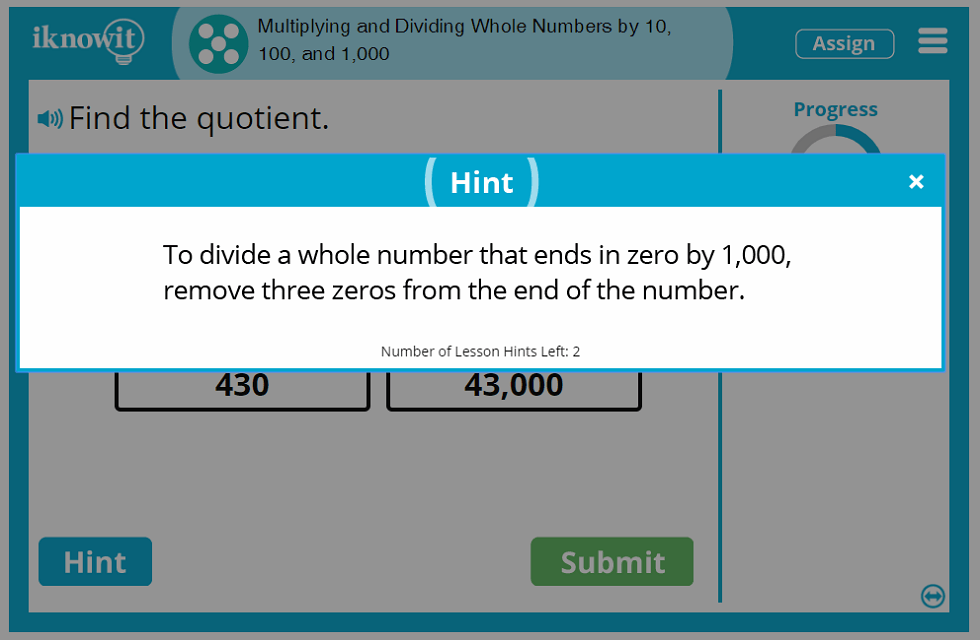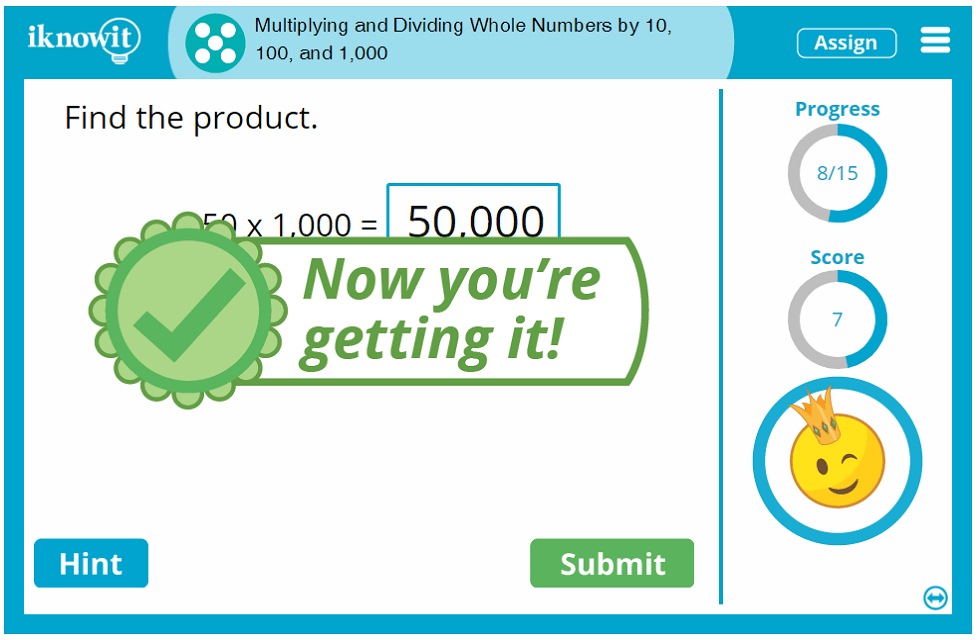## Why Choose 'I Know It'

Educators and students alike enjoy using the "I Know It" math program to supplement their classroom math curriculum. Educators appreciate the scope and variety of math topics we cover in our expanding program, as well as the quality of the lesson content. Students have fun going through their math practice and seeing the silly animated characters respond with a dance or a trick when they answer a question correctly. Plus, the positive reinforcement and opportunity to earn awards for their math milestones help children stay motivated to improve their skills.

We hope you will try out this fifth grade multiplication and division practice activity with your class! Be sure to check out the hundreds of other math topics available on "I Know It."

## 'I Know It' Membership Benefits

When you sign up for a free sixty-day trial of iKnowIt.com, you can try this math lesson – or any math lesson on our site – at no cost! In the free trial mode, students can play any math game on "I Know It," but they will be capped at a total of twenty-five questions per day across all math lessons. For unlimited access to our math program, you will need to become a paying member of the website.

We think you will love all the benefits that come with your "I Know It" membership! Here are a few examples: create a class roster and add your students to it; assign individual user names and passwords to all of your students; give different lesson assignments to each student based on their individual skill level; change lesson settings, such as limiting the hints or turning off the animated characters; and print, save, and email student progress reports.

Your students will find it easy and straightforward to use the student mode of "I Know It". When they log in with their unique user name and password, they will be shown a kid-friendly homepage where they can access all their assignments as well as explore additional math activities at their level or different levels. The grade levels in the student mode appear with alphabetical labels instead of numbers (Level A, Level B, Level C, and so on), making it easy for you discretely assign lessons based on skill level.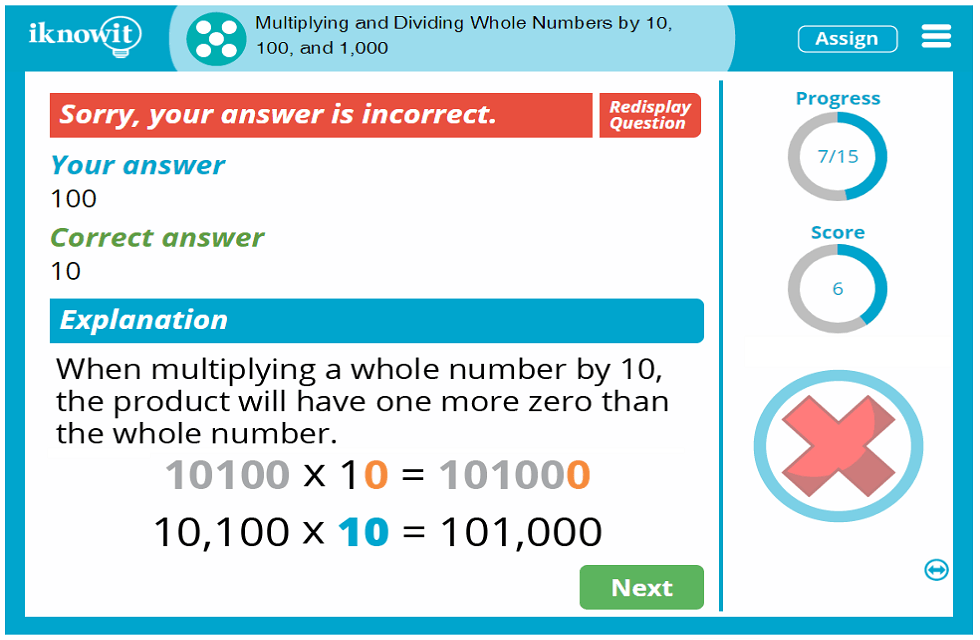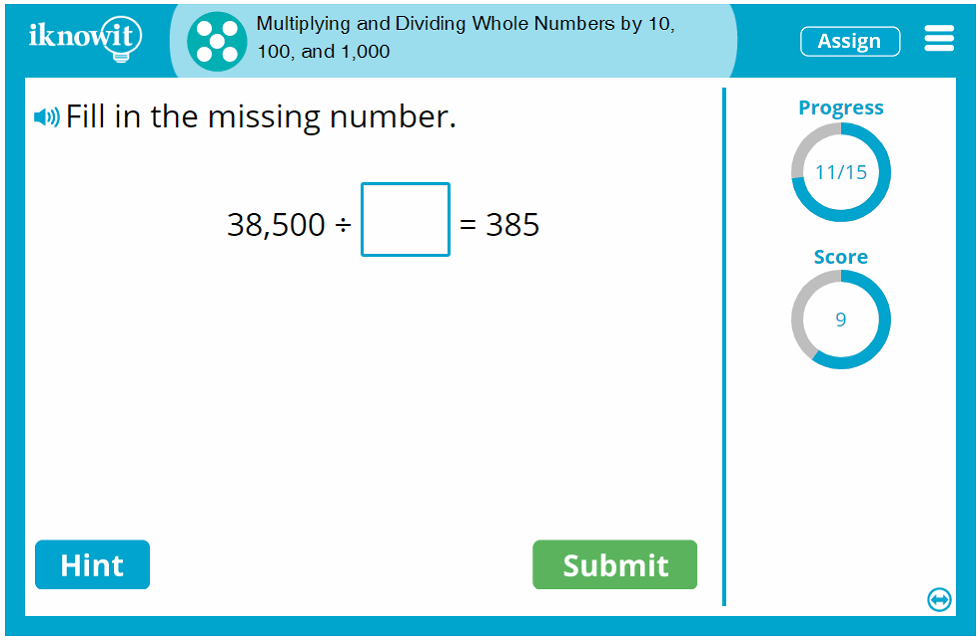## Level

This Level E mixed multiplication and division lesson may be ideal for fifth grade students.

## Common Core Standard

5.NBT.2
Number And Operations In Base Ten

Students will explain patterns in the number of zeroes of the product when multiplying a number by powers of ten, and explain patterns in the placement of the decimal point when a decimal is multiplied or divided by a power of ten.

## You might also be interested in...

Multiplying and Dividing Whole Numbers by Powers of Ten (Level E)
In this fifth grade-level math lesson, students will practice multiplying and dividing whole numbers by powers of ten. Questions are presented in drag-and-drop, multiple choice, and fill-in-the-blank format.

Multiplying and Dividing Decimals by Powers of Ten (Level E)
Students will practice multiplying and dividing decimals by powers of ten in this fifth grade-level math practice activity. Questions are given in various formats, including complete-the-table, multiple choice, and fill-in-the-blank.